# Line detection autopilot using Python + OpenCV.¶

Feb 2017

Line detection autopilot for DIYRobocars using OpenCV (python port of CompoundEye)

In a previous post I walked through how to create a lane keeping autopilot using an end-to-end neural network. This post shows how to create a lane keeping autopilot using line detection computer vision algorithms.

This is essentially a python port of the C++ computer vision autopilot, written by Haoyang Wang, and Jason Devitt from Compound Eye. It was the only vehicle to complete the first DIYRobocar Race.In :
import os
import cv2
import numpy as np
import random
import math

import matplotlib
from matplotlib.pyplot import imshow
from matplotlib import pyplot as plt
%matplotlib inline

In :
dir_path = '/home/wroscoe/donkey_data/sessions/wr_1240/'
images = os.listdir(dir_path)
img_paths = [os.path.join(dir_path, i) for i in images]
img_paths.sort()

#Read images, flip them vertically, and convert them to RGB color order
img_all = np.array([cv2.cvtColor(cv2.imread(p), cv2.COLOR_BGR2RGB) for p in img_paths])

#find image dimensions
imshow(img_all)

Out:
<matplotlib.image.AxesImage at 0x7fe445d4c978>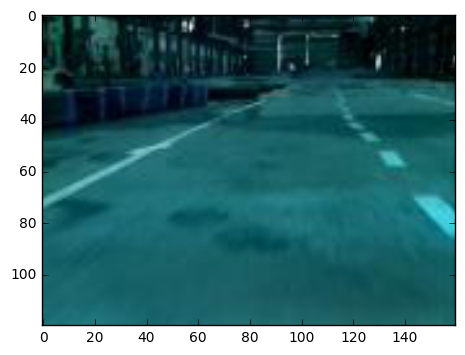A third of this pictures shows the warehouse rather than the track. Lets cut off this part of the image.

In :
img_all = np.array([img[40:, :] for img in img_all])
img_height = img_all.shape
img_width = img_all.shape

imshow(img_all)

Out:
<matplotlib.image.AxesImage at 0x7fe445d34470>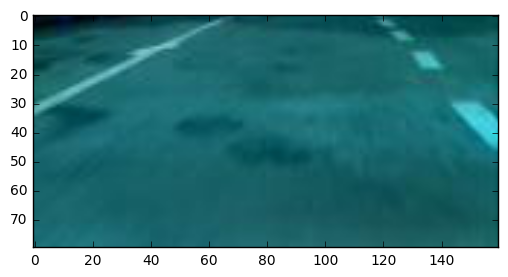In :
#only use a couple example images
img_arr = img_all[95:100]

In :
#helper function to show several images
def show_imgs(img_arr, cmap=None):

fig, ax = plt.subplots(1, img_arr.shape, figsize=(15, 6),

axoff = np.vectorize(lambda ax:ax.axis('off'))
axoff(ax)

for i, img in enumerate(img_arr):
ax[i].imshow(img, cmap=cmap)

In :
#show original images
show_imgs(img_arr)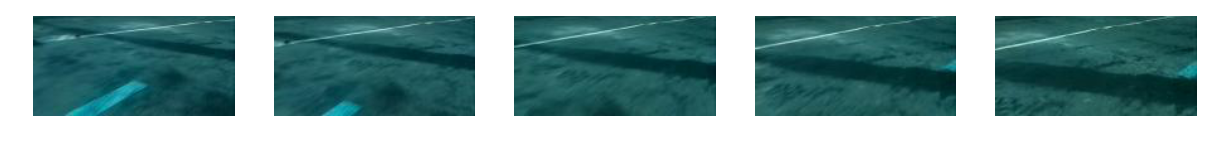## Find Lines¶

Now that we have our pictures loaded, lets find the lines of the course.

In :
#remove colors and show greyscale
gray_arr = np.array([cv2.cvtColor(img, cv2.COLOR_BGR2GRAY) for img in img_arr])
show_imgs(gray_arr, cmap='gray')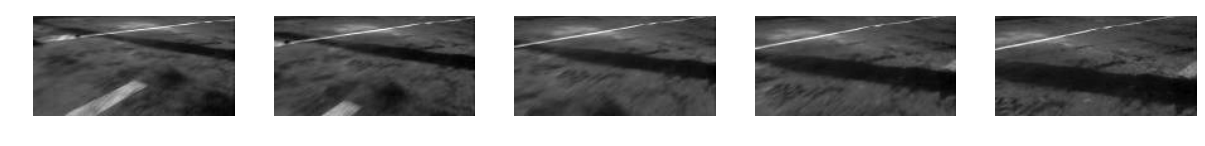In :
#blur images to avoid recognizing small lines
blur_arr = np.array([cv2.blur(arr,(5,5)) for arr in gray_arr])
#blur_arr = gray_arr
show_imgs(blur_arr, cmap='gray')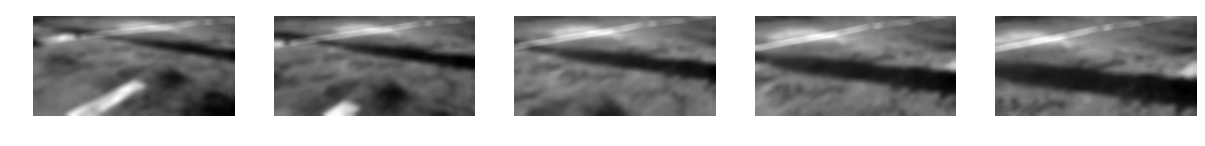In :
#use canny threshold to find edges of shapes
canny_threshold1 = 100
canny_threshold2 = 130

canny_arr = np.array([cv2.Canny(arr, canny_threshold1, canny_threshold2) for arr in blur_arr])
show_imgs(canny_arr, cmap='gray')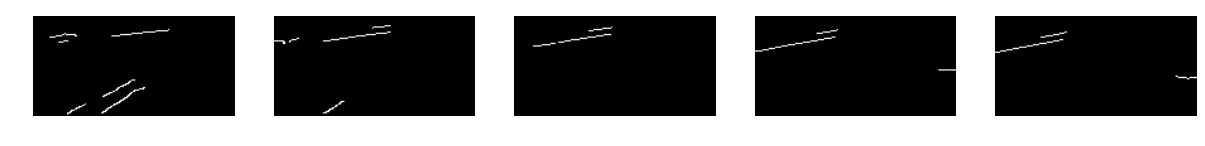In :
hough_threshold = 2
min_line_length = 3
max_gap = 5
rho = 2.
theta = .3

line_arr = []
line_coord_arr = []
line_count = 0
for i, canny in enumerate(canny_arr):
lines = cv2.HoughLinesP(canny, rho, theta, hough_threshold,
min_line_length, max_gap)
img = img_arr[i]
if lines is not None:
for line in lines:
#format line to be drawn
x1, y1, x2, y2 = line
line_coord = np.array([[[x1, y1], [x2, y2]]], dtype=float)

#draw line
cv2.line(img,(x1,y1),(x2,y2),(0,255,0),3)

line_count += 1

line_arr.append(img)

line_arr = np.array(line_arr)

show_imgs(line_arr)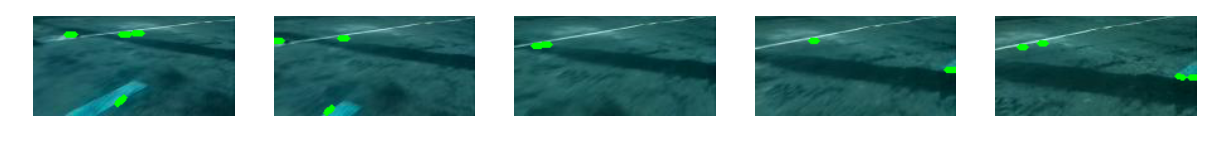## Get a birds eye view of the lines¶

To calculate the steering angle we'll use a perspective transform to simulate a birds eye view from the tip. This way we can calculate the actual angle of the line relative to the car.

The first step is to calculate the required transform from the camera angle to a top view. OpenCV provides an easy function to do this if you can provide a rectangle before and after the transform.

1. Find the corners of a rectangle on the road.
2. Define where those corners would be from a birds eye view.
3. Use OpenCV to find this transformation matrix.
4. Use this transformation matrix to change the perspective of your images.

I did this by first taking picture of a standard 8.5"x11" letter size paper from my car and finding the corner cordinates.

In :
#Load calibration image
img_path = '/home/wroscoe/donkey_data/sessions/cv_orient/frame_00001_ttl_0_agl_0_mil_0.jpg'

#coordinates of corners: order is [top left, top right, bottom right, bottom left]
corners = np.array([(57,39), (110,39), (122,75), (39, 75)], dtype="float32")

#draw points on new image
img2 = img.copy()
for c in corners:
cv2.circle(img2, tuple(c), 3, (0,255,0), -1)

imshow(img2)

Out:
<matplotlib.image.AxesImage at 0x7fe43efb9eb8>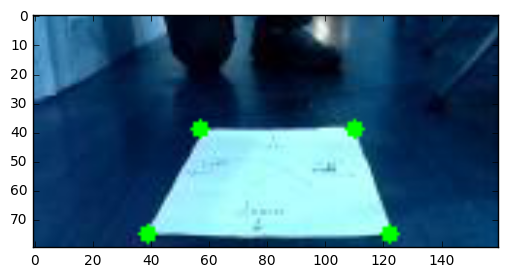Now we define where that rectangle should be if we're looking from the top veiew perspective and calculate the transformation matrix.

In :
def four_point_transform(pts):

maxWidth, maxHeight = 300, 300
hwratio = 11/8.5 #letter size paper
scale = int(maxWidth/12)

center_x = 150
center_y = 250

dst = np.array([
[center_x - scale, center_y - scale*hwratio], #top left
[center_x + scale, center_y - scale*hwratio], #top right
[center_x + scale, center_y + scale*hwratio], #bottom right
[center_x - scale, center_y + scale*hwratio], #bottom left
], dtype = "float32")

# compute the perspective transform matrix and then apply it
M = cv2.getPerspectiveTransform(pts, dst)

return M

M = four_point_transform(corners)
M

Out:
array([[  2.43902439e+00,   6.30081301e+00,  -6.15853659e+01],
[ -4.30211422e-15,   1.61246610e+01,  -6.61644977e+01],
[ -1.45283091e-17,   4.06504065e-02,   1.00000000e+00]])

When we apply that transformation to the same image we can see that the paper now looks like it would from the top.

In :
warped =  cv2.warpPerspective(img2, M, (300, 300))
imshow(warped)

Out:
<matplotlib.image.AxesImage at 0x7fe43f05aa58>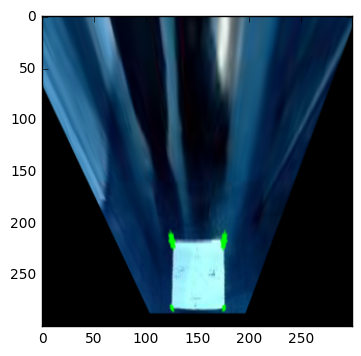Here is the same transformation applied to pictures from the track.

In :
warped_arr = np.array([cv2.warpPerspective(i, M, (300, 300)) for i in img_arr])
show_imgs(warped_arr)